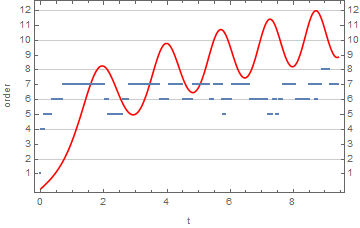# Preface

This section discusses numerical solutions to the differential equations of order larger than one.

# Numerical Solutions

The order of a numerical method used in NDSolve is a variable. Based on What's inside InterpolatingFunction[{{1., 4.}}, <>]?, the following shows the order at each step. Note that the first few steps are NDSolve getting its bearings before the first Adams steps (order 4). With InterpolationOrder -> All, the solution is returned with local series for the Adams steps. Their length should be one more than the order of the step, I think. The documentation says it should be the "same order as the underlying method used."

Example. let use Adams method to solve numerically the following initial value problem

adamssol = NDSolve[{x''[t] + x[t]^3 == 2*t, x == 0, x' == 0.5}, x, {t, 0, 3 $Pi]}, Method -> "Adams", InterpolationOrder -> All]; adamsifn = x /. First@adamssol ListPlot[{Transpose@{Flatten@adamsifn["Grid"], Map[Length, adamsifn[]] - 1}, (*ifn[]= local series coefficients*) Transpose@{Flatten@adamsifn["Grid"], Rescale[#, MinMax[#], {0, 12}] &@adamsifn["ValuesOnGrid"]}}, Joined -> {False, True}, Frame -> True, FrameLabel -> {"t", "order"}, FrameTicks -> {Automatic, Range@12}, GridLines -> {None, Automatic}, PlotStyle -> {Thick, Red}]Example: Rayleigh's equation is a non-linear equation \[ x'' + \mu \left( x^2 -1\right) x +x =0 ,$

that arises in the study of the motion of a violin string. We plot three solutions subject to three different initial conditions:

numsol = NDSolve[{x''[t] + ((1/3)*(x'[t])^2 - 1)*x'[t] + x[t] == 0, x == 1,
x' == 0}, x[t], {t, 0, 15}]
Out= {{x[t] -> InterpolatingFunction[{{0., 15.}}],<>],[t]}}
numsol1 = NDSolve[{x''[t] + ((1/3)*(x'[t])^2 - 1)*x'[t] + x[t] == 0, x == 1, x' == 0}, x[t], {t, 0, 15}]
Out= {{x[t] -> InterpolatingFunction[{{0., 15.}}],<>],[t]}}
numsol2 = NDSolve[{x''[t] + ((1/3)*(x'[t])^2 - 1)*x'[t] + x[t] == 0, x == 0.1, x' == 0}, x[t], {t, 0, 15}]
Out= {{x[t] -> InterpolatingFunction[{{0.,15.}}, <>][t]}}
To see three graphs, we type:
numsol3 = NDSolve[{x''[t] + ((1/3)*(x'[t])^2 - 1)*x'[t] + x[t] == 0, x == 0, x' == 2}, x[t], {t, 0, 15}]
Plot[Evaluate[x[t] /. {numsol, numsol2, numsol3}], {t, 0, 15},
PlotStyle -> {GrayLevel[0.1], Dashed, Thick}]

To identify each graph, it is helpful to use PlotLegend option:

Plot[Evaluate[x[t] /. {numsol, numsol2, numsol3}], {t, 0, 15}, PlotStyle -> {GrayLevel[0.1], Dashed, Thick},
PlotLegends -> Automatic]
Plot[Evaluate[x[t] /. {numsol, numsol2, numsol3}], {t, 0, 15}, PlotStyle -> {GrayLevel[0.1], Dashed, Thick},
PlotLegends -> {"IC: x(0)=1, x'(0)=0", "IC: x(0)=0.1, x'(0)=0", "IC: x(0)=0, x'(0)=2"}]
■

Example: Consider the second-order nonlinear differential equation with the product of the derivative and a sinusoidal nonlinearity of the solution

$\frac{{\text d}^2 y}{{\text d}x^2} - \cos \left( x\,y \right) \frac{{\text d}y}{{\text d}x} = x, \qquad y(0) =1, \quad y' (0) =-1.$
■
1. Quarteroni, Alfio, Numerical Models for Differential Problems,
2. , Springer International Publishing, 2017, New York, ISBN: 978-3-319-49316-9; doi: 10.1007/978-3-319-49316-9
3. Schwalbe, Dan, and Wagon, Stan, VisualDSolve, Springer-Verlag, New York, 1997, ISBN: 978-1-4612-7473-5